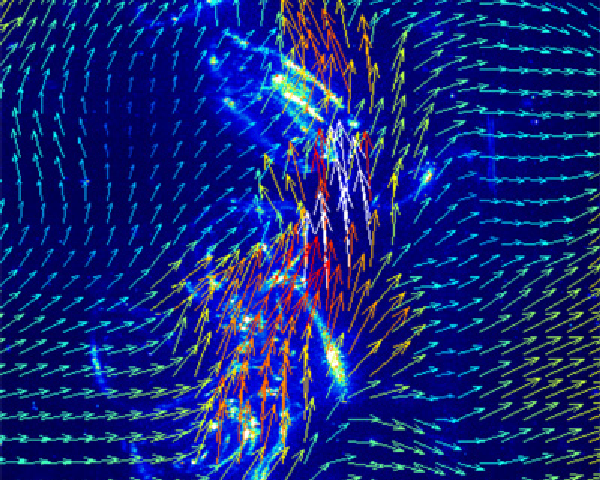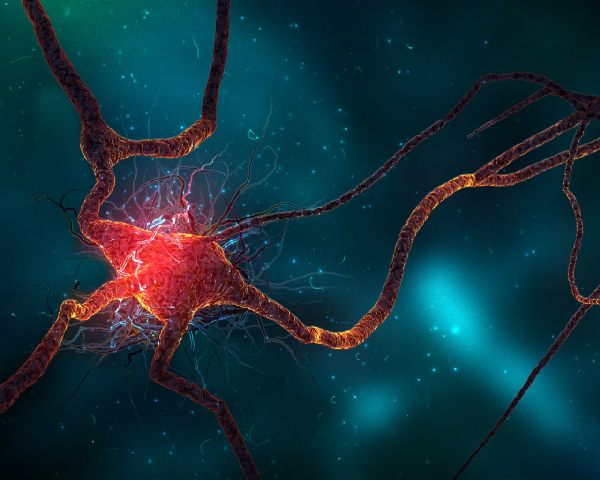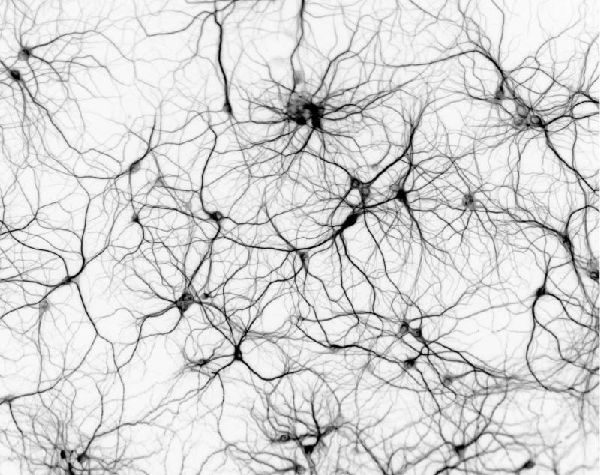easy offline web builder

### General objective

The scope of this summer school is to bring together 25-35 PhD students in quantitative life sciences and teach them a wide range of mathematical approaches to analysis and modeling in neuroscience. The topics cover a broad range of approaches in mathematical and computational neuroscience, and both single cell and network models will be covered.

## Description

### Overview

The workshop will be jointly organized with the Faculty of Science at the University of Copenhagen (Science), Dynamical Systems Interdiscliplinary Network (DSIN), the European Society for Mathematical and Theoretical Biology (ESMTB) and the European Mathematical Society (EMS) . This workshop fits into a series of annual workshops on mathematical biology organized with the EMS.

The mornings will start with lectures by the invited group of international experts. These lectures will showcase exemplary stories that have combined mathematical modeling and experimental neuroscience and discuss a number of mathematical methods in-depth. The lectures will cover a wide range of topics within the field. During the afternoons, there will be grouped hands-on sessions on selected problems raised and discussed during the lectures.

The topics will cover both single cell and network models, allowing you to obtain an overview of current practices and approaches to analyzing data in the field.

### Lecturers

The invited lecturers will cover a broad range of techniques in mathematical and computational neuroscience. In order to provide you with an outstanding quality of presentations, we have invited a group of distinguished international researchers: Professor of Applied Mathematics Stephen Coombes from University of Nottingham, expert in application of principles from nonlinear dynamics and statistical physics to the study of neural systems, Principal Investigator Alex Roxin from Centre Recerca Matematica, Barcelona, expert in Theoretical Neurobiology of Cortical Circuits, Professor in Probability Eva Löcherbach from Université Cergy-Pontoise, expert in stochastic processes and interacting graphs, and Professor Patricia Reynaud-Bouret from University of Nice, expert in point process models and statistics for spike trains and their dependence structure and Rune W. Berg from University of Copenhagen, an expert in functional neuronal networks and sensory and motor processing.

### Hands on experience

Signal processing of experimental data in Neuroscience

Topics will cover filters for processing experimental data, time-frequency analysis, and spike rate - theory and estimation. Introductory lectures will give a general introduction to analysis of electrical signals from the nervous system. You will alternate between lectures on the various topics and problem based learning. After this introduction we will use R and/or MATLAB to analyse and process data. There will be assignments to work on in groups or by yourself. The lecturers will be there to assist the you throughout the week.

Numerical experiments and simulation of stochastic models

You will play with methods to determine if synchronisation among spike trains is present or not. For this you will work with R and/or MATLAB to perform all statistical analysis. Also tutorials for numerically simulating coupled oscillator networks and neural field models will be introduced in the hands on sessions.

### Topics#### Dynamics of neural networks

The tools of dynamical systems theory are having an increasing impact on our understanding of patterns of neural activity. Some of the more popular single neuron models will be introduced and their behaviour in terms of bifurcation diagrams, phase-planes and phase-response curves will be explained.

For limit cycle oscillators, the coupled oscillator approach that has provided a framework for understanding behaviour in neural networks with weak synaptic and gap junction coupling will be reviewed. Then it will be shown how results for strong coupling can be obtained by focusing on a specific class of spiking neural models, namely (non-smooth) planar integrate-and-fire models.

It will be described how to build tractable tissue level models that maintain a strong link with biophysical reality. These models typically take the form of nonlinear integro-differential equations. Their non-local nature has led to the development of a set of analytical and numerical tools for the study of waves, bumps and patterns, based around natural extensions of those used for local differential equation models.

The relevance of neural field models for describing the brain at the large scales necessary for interpreting EEG and MEG data will be discussed. Recent results on next generation neural field models obtained via a mean field reduction from networks of nonlinear integrate-and-fire neurons will also be presented.#### Meanfield theory for networks of spiking neurons

If functional units in the brain are large networks then we may not need to know the detailed dynamics of each neuron, but rather just a measure of the mean activity. Can we develop such a meanfield theory for networks of model spiking neurons?

The by now classical meanfield theory for irregularly spiking neurons will be discussed, which is based on two main assumptions: 1 - neurons receive large numbers of weak, uncorrelated inputs and 2 - neurons are Poisson processes. This is a very general theory which works for many spiking neuron models, but which only allows us to calculate stationary states, their stability, and the response to weak inputs.

A complementary theory will also be discussed, which only works for quadratic integrate-and-fire neurons with quenched variability, but which allows us to derive exact equations for the mean-field. These equations capture the full nonlinear response of the network and is simple enough to allow for extensive analysis.#### Modeling interacting networks as processes with variable length

A class of recently introduced models to describe networks of neurons as stochastic processes with memory of variable length will be presented. These are non-Markovian processes in high or infinite dimension in which the past dependence of transition probabilities or intensities has a range that is finite but depends on the particular history.

Starting from existence results, we study related mean-field models in continuous time and their large population limits, and discuss the relation with associated Piecewise Deterministic Markov Processes (PDMP’s) and state results concerning their longtime behavior.

We will look at two important problems of statistical inference in such models: estimation of the spiking rate function and estimation of the neuronal interaction graph.#### Detection of dependence between neurons and synchronisation

Synchronisation is an important phenomenon in neuroscience, and this kind of dependency between neuronal activity may play an important role for how the brain encodes information. An essential mathematical/statistical question is therefore how to detect such phenomena in a setup where the observations are scarce, noisy and always fluctuating.

The Unitary Events methods will be described (first introduced by Grün in the 90's) which makes rigorous the distinction between synchronization and pure coincidence and how statistical testing procedures can distinguish between them.

The notion of local independence between the spiking activity of neurons and how estimating a graph of local independence can infer functional connectivity will be explained.